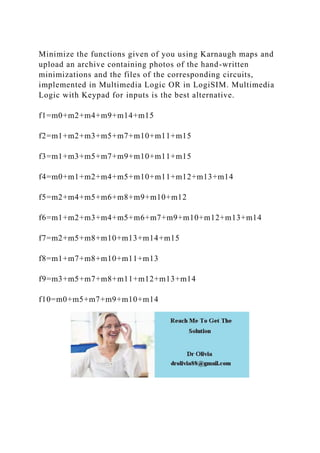Seu SlideShare está sendo baixado. ×

# Minimize the functions given of you using Karnaugh maps and upload a.docx

Anúncio
Anúncio
Anúncio
Anúncio
Anúncio
Anúncio
Anúncio
Anúncio
Anúncio
Anúncio
AnúncioCarregando em…3
×

1 de 1 Anúncio

# Minimize the functions given of you using Karnaugh maps and upload a.docx

Minimize the functions given of you using Karnaugh maps and upload an archive containing photos of the hand-written minimizations and the files of the corresponding circuits, implemented in Multimedia Logic OR in LogiSIM. Multimedia Logic with Keypad for inputs is the best alternative.

f1=m0+m2+m4+m9+m14+m15

f2=m1+m2+m3+m5+m7+m10+m11+m15

f3=m1+m3+m5+m7+m9+m10+m11+m15

f4=m0+m1+m2+m4+m5+m10+m11+m12+m13+m14

f5=m2+m4+m5+m6+m8+m9+m10+m12

f6=m1+m2+m3+m4+m5+m6+m7+m9+m10+m12+m13+m14

f7=m2+m5+m8+m10+m13+m14+m15

f8=m1+m7+m8+m10+m11+m13

f9=m3+m5+m7+m8+m11+m12+m13+m14

f10=m0+m5+m7+m9+m10+m14
.

Minimize the functions given of you using Karnaugh maps and upload an archive containing photos of the hand-written minimizations and the files of the corresponding circuits, implemented in Multimedia Logic OR in LogiSIM. Multimedia Logic with Keypad for inputs is the best alternative.

f1=m0+m2+m4+m9+m14+m15

f2=m1+m2+m3+m5+m7+m10+m11+m15

f3=m1+m3+m5+m7+m9+m10+m11+m15

f4=m0+m1+m2+m4+m5+m10+m11+m12+m13+m14

f5=m2+m4+m5+m6+m8+m9+m10+m12

f6=m1+m2+m3+m4+m5+m6+m7+m9+m10+m12+m13+m14

f7=m2+m5+m8+m10+m13+m14+m15

f8=m1+m7+m8+m10+m11+m13

f9=m3+m5+m7+m8+m11+m12+m13+m14

f10=m0+m5+m7+m9+m10+m14
.

Anúncio
Anúncio

### Minimize the functions given of you using Karnaugh maps and upload a.docx

1. 1. Minimize the functions given of you using Karnaugh maps and upload an archive containing photos of the hand-written minimizations and the files of the corresponding circuits, implemented in Multimedia Logic OR in LogiSIM. Multimedia Logic with Keypad for inputs is the best alternative. f1=m0+m2+m4+m9+m14+m15 f2=m1+m2+m3+m5+m7+m10+m11+m15 f3=m1+m3+m5+m7+m9+m10+m11+m15 f4=m0+m1+m2+m4+m5+m10+m11+m12+m13+m14 f5=m2+m4+m5+m6+m8+m9+m10+m12 f6=m1+m2+m3+m4+m5+m6+m7+m9+m10+m12+m13+m14 f7=m2+m5+m8+m10+m13+m14+m15 f8=m1+m7+m8+m10+m11+m13 f9=m3+m5+m7+m8+m11+m12+m13+m14 f10=m0+m5+m7+m9+m10+m14